# Symbols in Geometry

## Common Symbols Used in Geometry

Symbols save time and space when writing. Here are the most common geometrical symbols:

Symbol Meaning Example In Words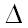Triangle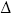ABC has 3 equal sides Triangle ABC has three equal sides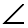Angle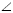ABC is 45° The angle formed by ABC is 45 degrees.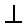Perpendicular AB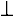CD The line AB is perpendicular to line CD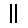Parallel EF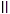GH The line EF is parallel to line GH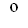Degrees 360° 360 degrees (a full rotation!)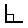Right Angle (90°)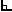is 90° A right angle is 90 degrees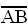Line Segment "AB" AB The line segment between A and B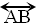Line "AB"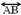The infinite line that includes A and B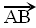Ray "AB"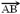The line that starts at A, goes through B and continues on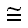Congruent (same shape and size)ABC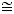DEF
Triangle ABC is congruent to triangle DEF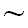Similar (same shape, different size)DEF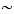MNO Triangle DEF is similar to triangle MNO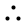Therefore a=bb=a a equals b, therefore b equals a

### Example: InABC,BAC isIs really saying: "In triangle ABC, the angle BAC is a right angle"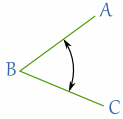### Naming Angles

For angles the central letter is where the angle is.

### Example:ABC is 45°

The point "B" is where the angle is.